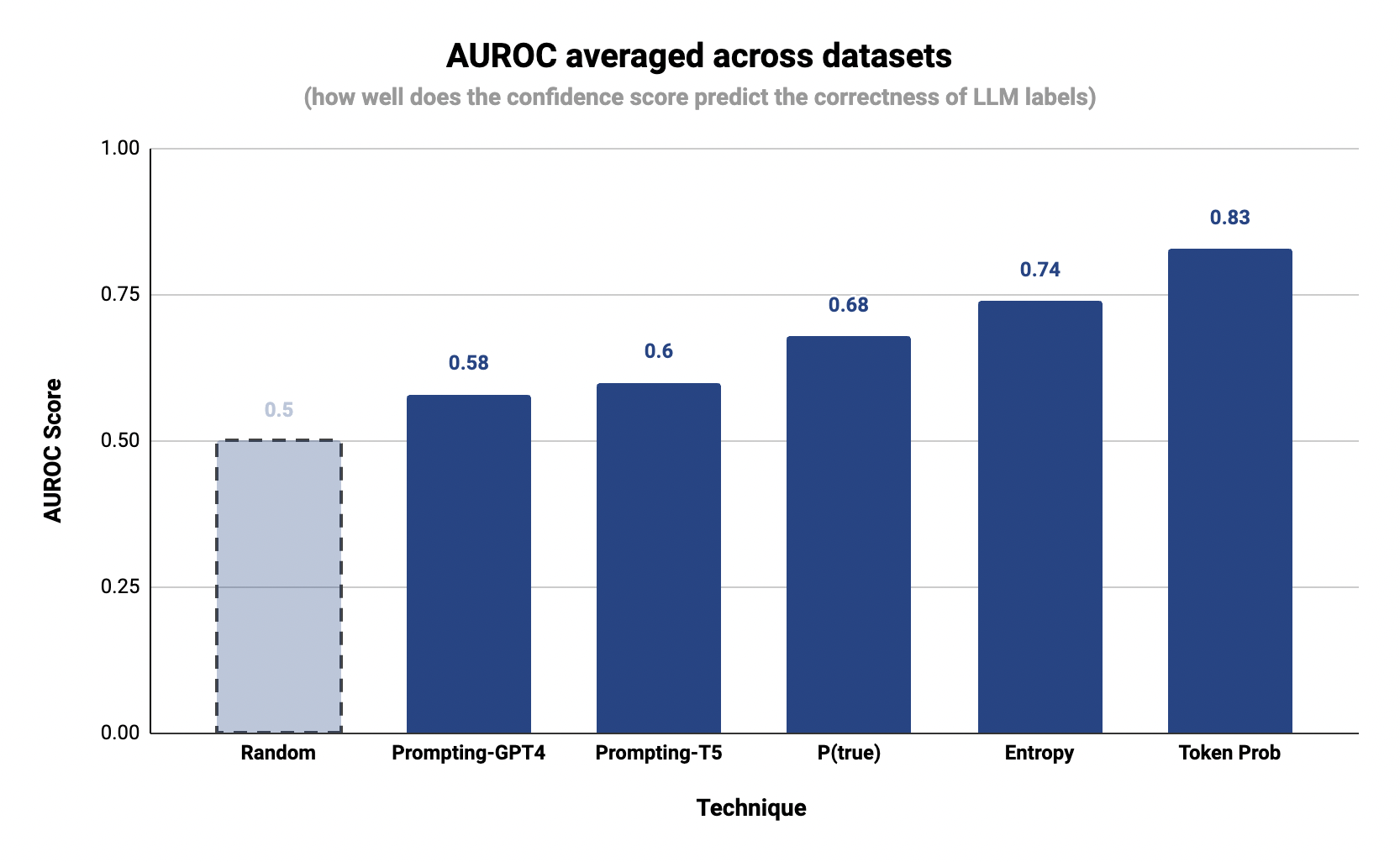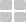# Labeling with ConfidenceBy
Dhruva Bansal, Nihit Desai## Summary

When leveraging LLMs for data labeling, it is important to be able to accurately estimate the model’s level of confidence in its own knowledge and reasoning. Doing so enables us to automatically reject low confidence labels and ensemble LLMs optimally. We examine different techniques for estimating confidence of LLM generated labels, in the context of data labeling for NLP tasks. Key takeaways:

• Token-level generation probabilities (commonly referred to as “logprobs”) are by far the most accurate technique for estimating LLM confidence.
• Explicitly prompting the LLM to output a confidence score, while popular, is highly unreliable. This technique had the lowest accuracy and the highest standard deviation across datasets. More often than not the LLM just hallucinates some number.

We used Autolabel, our recently open-sourced library, to run all the experiments that are a part of this report.

## Background & Motivation

Next generation data labeling tools like Autolabel leverage LLMs to create large, diverse labeled datasets. In an application domain like labeling where correctness is critical, it is imperative to be able to accurately estimate the model’s level of confidence in its own knowledge and reasoning. Doing so enables us to automatically reject low confidence labels, ensemble LLMs optimally, and learn more about strengths and weaknesses of any given LLM.

As a motivating example, consider the Toxic comments classification dataset from our labeling benchmark. If we are able to estimate the LLM’s confidence level alongside the labels it generates, we can calibrate the model’s label quality (% agreement with ground truth labels) at any given confidence score. We can then decide an operating point (confidence threshold) for the LLM, and reject all labels below this threshold.

This blog post summarizes our learnings around estimating confidence of LLM generated labels. We demonstrate the efficacy of four different confidence estimation techniques across a wide range of text labeling tasks/datasets.

## Methodology

####  Setup

1. For an input x, we generate a label y by prompting the Labeling LLM (GPT-4). The setup and prompts for doing this follows exactly the methodology described in the LLM data labeling benchmark
2. Next, we estimate the confidence c, given x and y using a Verifier LLM (FLAN-T5-XXL or GPT-4). This score quantifies the Verifier LLM’s confidence in the given label y being correct. We describe four confidence calculation methods that we benchmark in the section below.
3. Then, we compare y with the ground truth label gt to decide whether the prediction is a “true positive” (y == gt) or “false positive” (y != gt).
4. Finally, we compute AUROC (Area Under the Receiver Operating Characteristic) to understand how “good” a specific confidence estimation method is. For building the ROC curve, we first compute true positive rate and false positive rates at various score thresholds. We use all distinct values of c as thresholds.

For generating the labels themselves, we use GPT-4 as the labeling LLM in this evaluation. Ideally we’d like to use the same LLM for verification as well, but token-level generation probabilities (logprobs) are currently not available for OpenAI chat engine models (including GPT-4). Hence we use FLAN-T5-XXL as the verifier LLM. For techniques that don’t rely on logprobs, we also report the AUROC numbers with GPT-4 as the verifier LLM.

####  Metric

The AUROC is a scalar value that summarizes the overall performance of the classifier by measuring the area under the ROC curve. It provides a measure of the classifier's ability to distinguish between the positive and negative classes across all possible classification thresholds. The confidence score that we are calculating can be thought of as a binary classifier where the labels are whether the model got a specific label correct (true positive) or incorrect (false positive). Using the plot above as a reference, we can see that a random classifier would have an AUROC of 0.5.

####  Datasets

We included the following datasets for this evaluation. These datasets are available for download here.

####  Techniques

We benchmark the following four methods for confidence estimation:

##### P(True):

The labeling LLM (GPT-4) generates a label (llm_label) given an input prompt. Using this, we prompt the verifier LLM to complete the following sentence. The token generation probability of “Yes” is used as the confidence score

##### Prompting for Confidence Score:

The labeling LLM (GPT-4) generates a label (llm_label) given an input (prompt). Using this, we prompt the verifier LLM to complete the following sentence. The value output by the verifier LLM is parsed as a float and used as the confidence score. If parsing is unsuccessful, we give the sample a confidence score of 0.0 by default.

##### Token probabilities:

For classification-like and QA tasks, this is simply the probability of the first token in the generated label output produced. For NER, probabilities are first generated for all tokens and then the probability of tokens for each entity is averaged to compute confidence scores per entity.

We use the Verifier LLM for estimating token probabilities of a prediction - we first generate the prediction logits for all tokens in the vocabulary, for the length of the output sequence. Then, we compute the softmax over the token probability distribution for each index and use the probabilities corresponding to respective tokens in the prediction as token probabilities.

##### Entropy:
• Exhaustive Entropy: This method is used to calculate entropy for classification-like tasks. First, we calculate the probability of each of the possible labels and then calculate the 2-bit shannon entropy of the probability distribution over possible labels.
• Semantic Entropy: This method is used to calculate entropy for generation-like tasks. We prompt the LLM to produce N predictions at a temperature of 0.5 and then group them using a pairwise ROGUE score. Then, we will calculate the average probability of each group of predictions and calculate the entropy of that prediction distribution.

## Results

As explained in the previous section, we evaluated the performance of five different confidence estimation techniques across different NLP tasks and datasets.

Takeaways:

1. Token probability is still by far the most accurate and reliable technique for estimating LLM confidence. Across all the 4 datasets, this technique achieves the highest AUROC (0.832), and the lowest standard deviation (+/- 0.07).
2. Explicitly prompting the LLM to output a confidence score is the least accurate. This technique had the lowest AUROC (0.58) and the highest standard deviation  (+/- 0.13) across datasets.  More often than not the LLM just hallucinates some number.
3. Even with token probabilities, we do see some variability in how well it can work for a given labeling task. From early exploration internally with public and proprietary datasets, we have found that fine tuning the verifier LLM on the target labeling task improves the calibration significantly. We hope to share more details about this exploration in a future blog post.

Here’s a breakdown of the performance by dataset:

## Qualitative Evaluation

In order to get a more intuitive understanding for which kinds of inputs LLMs are more confident, vs less confident about, here’s what we did:

1. Compute embeddings for all inputs in the dataset using sentence transformers
2. Project all inputs into a 2D embedding map using UMAP
3. Overlay this projection map with useful metadata such as confidence score and “whether the LLM label is correct i.e. matches ground truth label” for each point.

We illustrate this with the Banking complaints classification dataset below. As you can see, clusters of low confidence inputs correspond quite well to incorrect LLM labels

Further, let’s examine a few rows with low confidence LLM labels (<= 0.2). Many of these inputs are ambiguous, and it is hard even for a human annotator to tell which of the labels (the one provided as ground truth in the dataset, or the LLM generated one) is “correct”.

## Estimating Confidence with Autolabel

Autolabel library relies on token level generation probabilities to estimate LLM label confidence. Generating confidence scores alongside labels is a simple config change - setting the key compute_confidence = True should initiate confidence score computation: